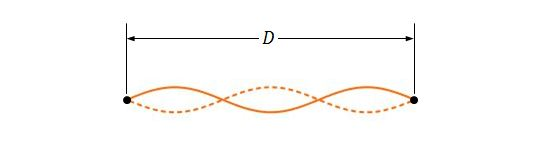Classical Mechanics

# Boundary conditions of standing waves

If two adjacent resonant frequencies are $440$ and $330\text{ Hz}$ for a string which is stretched to a length of $77.0\text{ cm},$ what is the lowest resonant frequency?

Consider a wire with length $8.0\text{ m}$ and mass $110\text{ g}$ which is under a tension of $290\text{ N}.$ What are the lowest and the third lowest frequency for a standing wave on the wire?

Consider two identical sinusoidal waves with the same wavelength and amplitude traveling in opposite directions along a string with a speed of $30.0 \text{ cm/s}.$ If the time interval between adjacent instants when the string is flat is $0.100\text{ s},$ what is the wavelength of the waves?

If a string with length $5.50\text{ m},$ mass $0.120\text{ kg},$ and tension $98.0\text{ N}$ is oscillating, approximately what are the longest possible wavelength and frequency for a standing wave?Suppose that a string, two ends of which is fixed at a distance of $D=120.0\text{ cm},$ has a linear density of $7.4\text{ g/m}$ and is under a tension of $150\text{ N}.$ If the string is oscillating in a standing wave pattern, as shown in the above figure, what is the approximate frequency of the traveling waves?

×